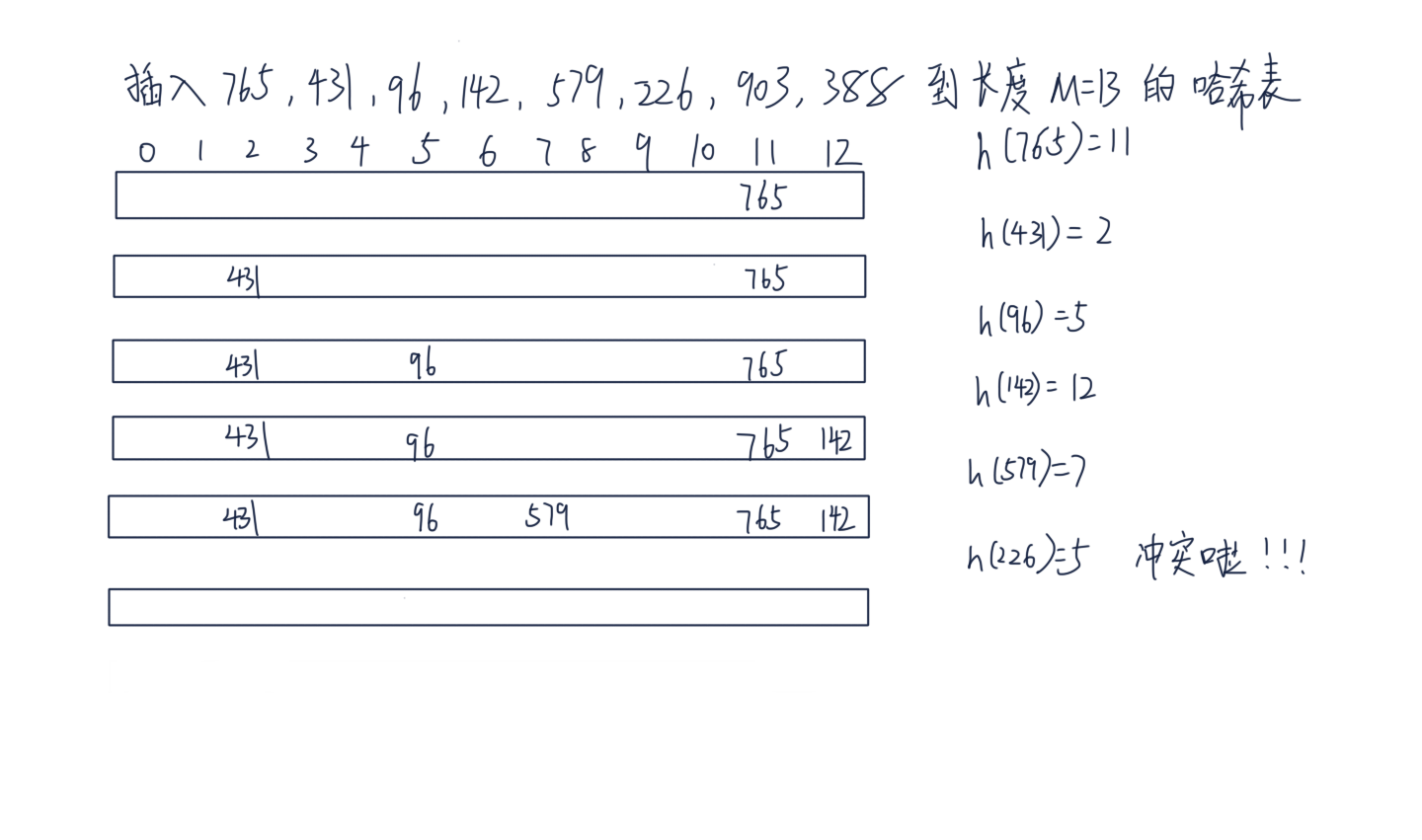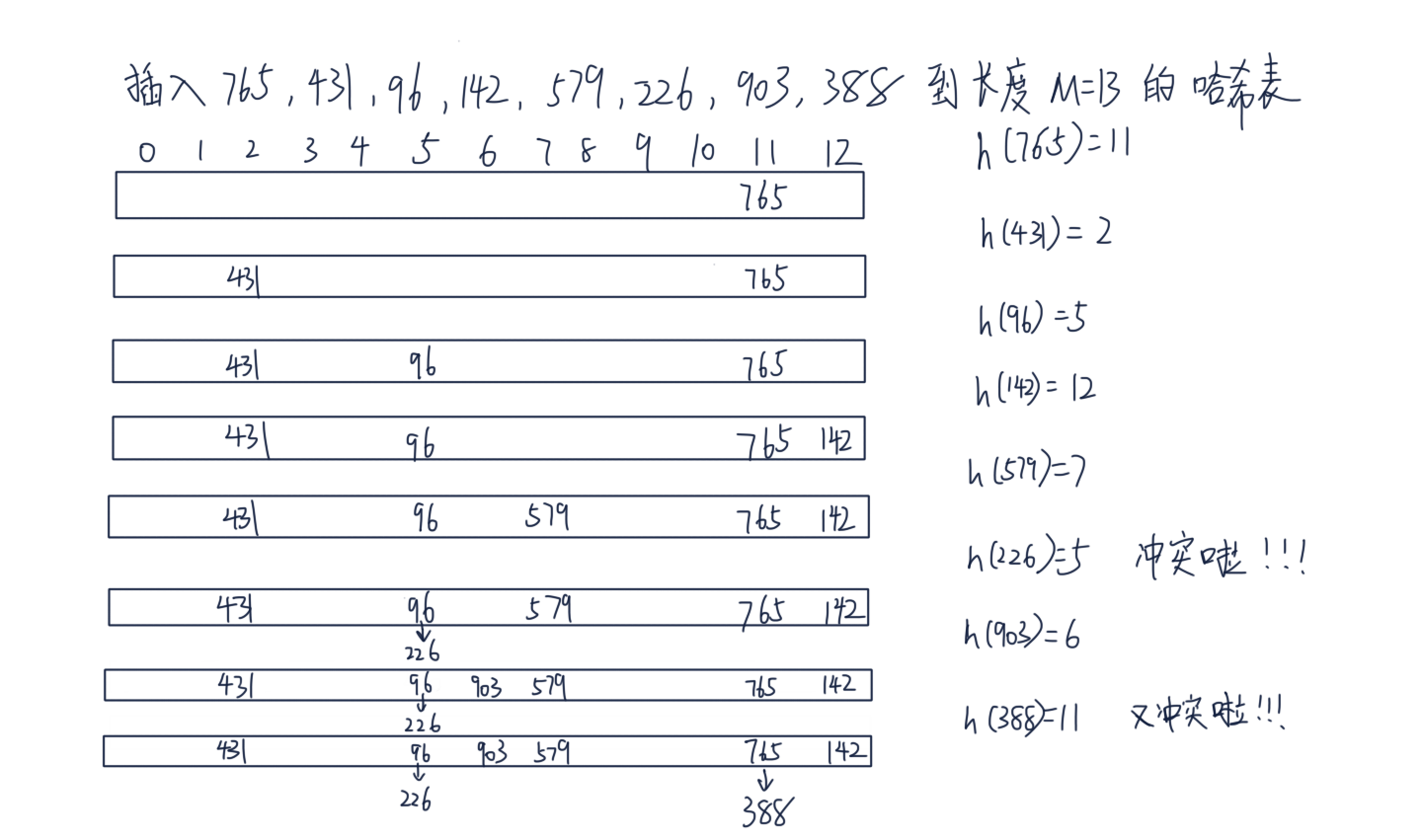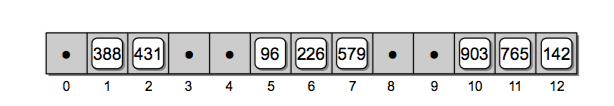# 哈希表的工作过程

h(key) = key % M


765, 431, 96, 142, 579, 226, 903, 388

M = 13
h(765) = 765 % M = 11
h(431) = 431 % M = 2
h(96) = 96 % M = 5
h(142) = 142 % M = 12
h(579) = 579 % M = 7
h(226) = 226 % M = 5
h(903) = 903 % M = 6
h(388) = 388 % M = 11# 哈希冲突 (collision)• 线性探查(linear probing): 当一个槽被占用，找下一个可用的槽。 $h(k, i) = (h^\prime(k) + i) \% m, i = 0,1,...,m-1$
• 二次探查(quadratic probing): 当一个槽被占用，以二次方作为偏移量。 $h(k, i) = (h^\prime(k) + c_1 + c_2i^2) \% m , i=0,1,...,m-1$
• 双重散列(double hashing): 重新计算 hash 结果。 $h(k,i) = (h_1(k) + ih_2(k)) \% m$

inserted_index_set = set()
M = 13

def h(key, M=13):
return key % M

to_insert = [765, 431, 96, 142, 579, 226, 903, 388]
for number in to_insert:
index = h(number)
first_index = index
i = 1
while index in inserted_index_set:   # 如果计算发现已经占用，继续计算得到下一个可用槽的位置
print('\th({number}) = {number} % M = {index} collision'.format(number=number, index=index))
index = (first_index +  i*i) % M   # 根据二次方探查的公式重新计算下一个需要插入的位置
i += 1
else:
print('h({number}) = {number} % M = {index}'.format(number=number, index=index))


h(765) = 765 % M = 11
h(431) = 431 % M = 2
h(96) = 96 % M = 5
h(142) = 142 % M = 12
h(579) = 579 % M = 7
h(226) = 226 % M = 5 collision
h(226) = 226 % M = 6
h(903) = 903 % M = 6 collision
h(903) = 903 % M = 7 collision
h(903) = 903 % M = 10
h(388) = 388 % M = 11 collision
h(388) = 388 % M = 12 collision
h(388) = 388 % M = 2 collision
h(388) = 388 % M = 7 collision
h(388) = 388 % M = 1# Cpython 如何解决哈希冲突

The first half of collision resolution is to visit table indices via this
recurrence:

j = ((5*j) + 1) mod 2**i

For any initial j in range(2**i), repeating that 2**i times generates each
int in range(2**i) exactly once (see any text on random-number generation for
proof).  By itself, this doesn't help much:  like linear probing (setting
j += 1, or j -= 1, on each loop trip), it scans the table entries in a fixed
order.  This would be bad, except that's not the only thing we do, and it's
actually *good* in the common cases where hash keys are consecutive.  In an
example that's really too small to make this entirely clear, for a table of
size 2**3 the order of indices is:

0 -> 1 -> 6 -> 7 -> 4 -> 5 -> 2 -> 3 -> 0 [and here it's repeating]


# 重哈希(Rehashing)

• get(key, default)
• remove(key)
class Slot(object):
"""定义一个 hash 表 数组的槽
注意，一个槽有三种状态，看你能否想明白
1.从未使用 HashMap.UNUSED。此槽没有被使用和冲突过，查找时只要找到 UNUSED 就不用再继续探查了
2.使用过但是 remove 了，此时是 HashMap.EMPTY，该探查点后边的元素扔可能是有key
3.槽正在使用 Slot 节点
"""
def __init__(self, key, value):
self.key, self.value = key, value

class HashTable(object):
pass


# 思考题

• 请你分析下哈希表插入和删除元素的平均时间复杂度是多少？我们都实现代码了，相信这个问题你可以回答上来
• Slot 在二次探查法里为什么不能直接删除？为什么我们要给它定义几个状态？

# 延伸阅读

• 《Data Structures and Algorithms in Python》11 章 Hash Tables
• 《算法导论》第三版 11 章散列表，了解几种哈希冲突的解决方式，以及为什么我们选择二次探查而不是线性探查法？
• 介绍 c 解释器如何实现的 python dict对象：Python dictionary implementation
• Python hash function implement